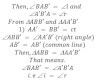# Laws Of Reflection Of Light According To The Wave Theory

The following are the laws of reflection of light.
1) The angle of incidence is equal to angle of reflection i.e. <i=<r.
2) The incident ray, reflected ray and normal lie on the same plane.

Proof:
Consider a plane wave front AB is incidenting towards the reflecting surface XY making the angle of incidence ‘i’. The perpendicular line is drawn to this wave front ie. a, b and c are incidenting rays According to the Huygen’s principle, every point of the wave front acts as source of secondary wavelets. Hence the point B of wave front AB forms the secondary wavelets at any time ’t’ whose surface just touches the point ‘B’ of reflecting surface XY. Such that BB’= ct where c is the velocity of light. At the sometime the point A of AB forms a secondary wavelets such that AA’= ct another points of wave front AB form the secondary wavelets such that the sum of the tangents drawn to their wavelets gives the new position of wave front i e. A ‘B’ is the reflected wave front which makes angle of reflection ‘r’. The perpendicular line drawn to this wave front i.e. a’, b’ and c’ are reflected ray .Since i and r are angle of incidence and angle of reflection.This proves the 1st law of reflection of light.

Since, incident wave front, reflecting surface and reflected wave front are perpendicular to the plane of paper. Then incident ray. Normal and reflected ray, which are perpendicular to the incident wave front, reflecting surface and reflected wave front are parallel to the plane of paper. Hence they lie on some plane. This proves the laws of reflection of light.